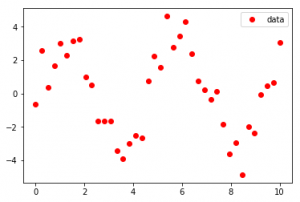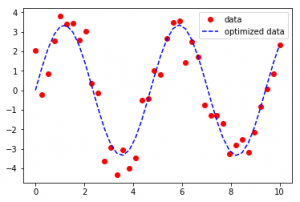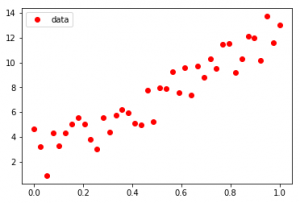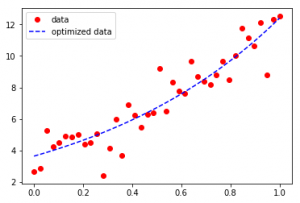GFG App
Open AppBrowser
Continue

Given a Dataset comprising of a group of points, find the best fit representing the Data.
We often have a dataset comprising of data following a general path, but each data has a standard deviation which makes them scattered across the line of best fit. We can get a single line using curve-fit() function.
Using SciPy :
Scipy is the scientific computing module of Python providing in-built functions on a lot of well-known Mathematical functions. The scipy.optimize package equips us with multiple optimization procedures. A detailed list of all functionalities of Optimize can be found on typing the following in the iPython console:

`help(scipy.optimize)`

Among the most used are Least-Square minimization, curve-fitting, minimization of multivariate scalar functions etc.
Curve Fitting Examples –
Input :Output :Input :Output :As seen in the input, the Dataset seems to be scattered across a sine function in the first case and an exponential function in the second case, Curve-Fit gives legitimacy to the functions and determines the coefficients to provide the line of best fit.

Code showing the generation of the first example –

## Python3

 `import` `numpy as np`   `# curve-fit() function imported from scipy` `from` `scipy.optimize ``import` `curve_fit`   `from` `matplotlib ``import` `pyplot as plt`   `# numpy.linspace with the given arguments` `# produce an array of 40 numbers between 0` `# and 10, both inclusive` `x ``=` `np.linspace(``0``, ``10``, num ``=` `40``)`     `# y is another array which stores 3.45 times` `# the sine of (values in x) * 1.334. ` `# The random.normal() draws random sample ` `# from normal (Gaussian) distribution to make` `# them scatter across the base line` `y ``=` `3.45` `*` `np.sin(``1.334` `*` `x) ``+` `np.random.normal(size ``=` `40``)`   `# Test function with coefficients as parameters` `def` `test(x, a, b):` `    ``return` `a ``*` `np.sin(b ``*` `x)`   `# curve_fit() function takes the test-function` `# x-data and y-data as argument and returns ` `# the coefficients a and b in param and` `# the estimated covariance of param in param_cov` `param, param_cov ``=` `curve_fit(test, x, y)`     `print``(``"Sine function coefficients:"``)` `print``(param)` `print``(``"Covariance of coefficients:"``)` `print``(param_cov)`   `# ans stores the new y-data according to ` `# the coefficients given by curve-fit() function` `ans ``=` `(param[``0``]``*``(np.sin(param[``1``]``*``x)))`   `'''Below 4 lines can be un-commented for plotting results ` `using matplotlib as shown in the first example. '''`   `# plt.plot(x, y, 'o', color ='red', label ="data")` `# plt.plot(x, ans, '--', color ='blue', label ="optimized data")` `# plt.legend()` `# plt.show()`

Output:

```Sine function coefficients:
[ 3.66474998  1.32876756]
Covariance of coefficients:
[[  5.43766857e-02  -3.69114170e-05]
[ -3.69114170e-05   1.02824503e-04]]```

Second example can be achieved by using the numpy exponential function shown as follows:

## Python3

 `x ``=` `np.linspace(``0``, ``1``, num ``=` `40``)`   `y ``=` `3.45` `*` `np.exp(``1.334` `*` `x) ``+` `np.random.normal(size ``=` `40``)`   `def` `test(x, a, b):` `    ``return` `a``*``np.exp(b``*``x)`   `param, param_cov ``=` `curve_fit(test, x, y)`

However, if the coefficients are too large, the curve flattens and fails to provide the best fit. The following code explains this fact:

## Python3

 `import` `numpy as np` `from` `scipy.optimize ``import` `curve_fit`   `from` `matplotlib ``import` `pyplot as plt`   `x ``=` `np.linspace(``0``, ``10``, num ``=` `40``)`   `# The coefficients are much bigger.` `y ``=` `10.45` `*` `np.sin(``5.334` `*` `x) ``+` `np.random.normal(size ``=` `40``)`   `def` `test(x, a, b):` `    ``return` `a ``*` `np.sin(b ``*` `x)`   `param, param_cov ``=` `curve_fit(test, x, y)`   `print``(``"Sine function coefficients:"``)` `print``(param)` `print``(``"Covariance of coefficients:"``)` `print``(param_cov)`   `ans ``=` `(param[``0``]``*``(np.sin(param[``1``]``*``x)))`   `plt.plot(x, y, ``'o'``, color ``=``'red'``, label ``=``"data"``)` `plt.plot(x, ans, ``'--'``, color ``=``'blue'``, label ``=``"optimized data"``)` `plt.legend()` `plt.show()`

Output:

```Sine function coefficients:
[ 0.70867169  0.7346216 ]
Covariance of coefficients:
[[ 2.87320136 -0.05245869]
[-0.05245869  0.14094361]]```
` `

The blue dotted line is undoubtedly the line with best-optimized distances from all points of the dataset, but it fails to provide a sine function with the best fit.
Curve Fitting should not be confused with Regression. They both involve approximating data with functions. But the goal of Curve-fitting is to get the values for a Dataset through which a given set of explanatory variables can actually depict another variable. Regression is a special case of curve fitting but here you just don’t need a curve that fits the training data in the best possible way(which may lead to overfitting) but a model which is able to generalize the learning and thus predict new points efficiently.

My Personal Notes arrow_drop_up